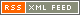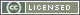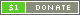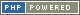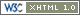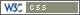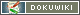# Mathematical Operators

Mathematical Operators are so named to distinguish them from basic arithmetic Operators, which are of course also essentially mathematical.

Symbol Type Name Description Example
Number
abs Prefix Absolute value Gives the positive numerical value of the term ` abs a `
sin Prefix Sine Gives the sine of the term, with the term in radians ` sin a `
cos Prefix Cosine Gives the cosine of the term, with the term in radians ` cos a `
tan Prefix Tangent Gives the tangent of the term, with the term in radians ` tan a `
log Prefix Logarithm Gives the base-10 logarithm of the term ` log a `
ln Prefix Natural logarithm Gives the natural logarithm of the term ` ln a `